# Direction Cosines

## Basic Definitions and Relations

Here we follow the notation of MF53.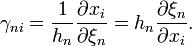$\gamma_{ni} = \frac{1}{h_n} \frac{\partial x_i}{\partial \xi_n} = h_n \frac{\partial\xi_n}{\partial x_i} .$

This means that the following inverse relationship applies in general: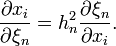$\frac{\partial x_i}{\partial \xi_n} = h_n^2 \frac{\partial\xi_n}{\partial x_i} .$

The coordinate system 123) is orthogonal if all the direction cosines obey the following relation:

DC.01

General Orthogonality Condition

 ∑ γmsγns = δmn, s

where the Kronecker delta function, δmn, is defined such that δmn = 1 if m = n but δmn = 0 if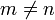$m \ne n$.

## Usage

### Scale Factors

The above relations can be used to define the scale factors (h1,h2,h3). For example,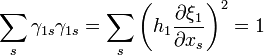$\sum_s \gamma_{1s}\gamma_{1s} = \sum_s \biggl( h_1 \frac{\partial\xi_1}{\partial x_s} \biggr)^2 = 1$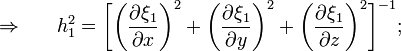$\Rightarrow ~~~~~ h_1^2 = \biggl[ \biggl(\frac{\partial\xi_1}{\partial x} \biggr)^2 + \biggl(\frac{\partial\xi_1}{\partial y} \biggr)^2 + \biggl(\frac{\partial\xi_1}{\partial z} \biggr)^2 \biggr]^{-1} ;$

or,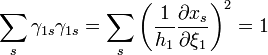$\sum_s \gamma_{1s}\gamma_{1s} = \sum_s \biggl( \frac{1}{h_1} \frac{\partial x_s}{\partial\xi_1} \biggr)^2 = 1$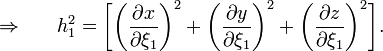$\Rightarrow ~~~~~ h_1^2 = \biggl[ \biggl(\frac{\partial x}{\partial\xi_1} \biggr)^2 + \biggl(\frac{\partial y}{\partial\xi_1} \biggr)^2 + \biggl(\frac{\partial z}{\partial\xi_1} \biggr)^2 \biggr] .$

### Unit Vectors

Direction cosines can be used to switch between the basis vectors of different orthogonal coordinate systems. The defining expressions are: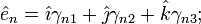$\hat{e}_n = \hat\imath \gamma_{n1} + \hat\jmath \gamma_{n2} + \hat{k}\gamma_{n3} ;$

and,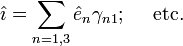$\hat\imath = \sum_{n=1,3}\hat{e}_n \gamma_{n1} ; ~~~~\mathrm{etc.}$

More explicitly, this last expression(s) implies,$\hat\imath$ =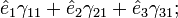$\hat{e}_1 \gamma_{11} + \hat{e}_2 \gamma_{21} + \hat{e}_3 \gamma_{31} ;$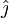$\hat\jmath$ =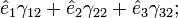$\hat{e}_1 \gamma_{12} + \hat{e}_2 \gamma_{22} + \hat{e}_3 \gamma_{32} ;$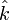$\hat{k}$ =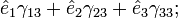$\hat{e}_1 \gamma_{13} + \hat{e}_2 \gamma_{23} + \hat{e}_3 \gamma_{33} ;$

notice that we have liberally used the idea that, for orthogonal systems, γnm = γmn.

### Orthogonality

How can we check to make sure that the coordinate ξ1 is everywhere orthogonal to the coordinate ξ2? Well, for an orthogonal system, the unit vectors should be everywhere perpendicular to one another, that is, the dot product of two (different) unit vectors should be zero at all coordinate positions. Drawing on the above unit-vector transformation expressions, this means that, for$m \ne n$,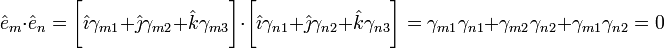$\hat{e}_m \cdot \hat{e}_n = \biggl[ \hat\imath \gamma_{m1} + \hat\jmath \gamma_{m2} + \hat{k}\gamma_{m3} \biggr] \cdot \biggl[ \hat\imath \gamma_{n1} + \hat\jmath \gamma_{n2} + \hat{k}\gamma_{n3} \biggr] = \gamma_{m1}\gamma_{n1} + \gamma_{m2}\gamma_{n2} + \gamma_{m1}\gamma_{n2} = 0$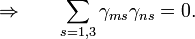$\Rightarrow ~~~~~ \sum_{s=1,3} \gamma_{ms}\gamma_{ns} = 0 .$

This is precisely the condition enforced on direction cosines in conjunction with their definition, shown above as Equation DC.01. Notice as well that, when m = n, Equation DC.01 is equivalent to the statement,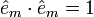$\hat{e}_m\cdot \hat{e}_m = 1$.

Here we'll illustrate how orthogonality can be checked for any axisymmetric coordinate system; that is, we'll examine behavior only in the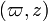$(\varpi,z)$ plane. First, note that,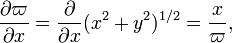$\frac{\partial\varpi}{\partial x} = \frac{\partial}{\partial x} (x^2 + y^2)^{1/2} = \frac{x}{\varpi} ,$

and,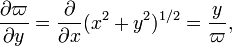$\frac{\partial\varpi}{\partial y} = \frac{\partial}{\partial x} (x^2 + y^2)^{1/2} = \frac{y}{\varpi} ,$

Hence,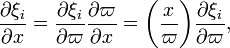$\frac{\partial\xi_i}{\partial x} = \frac{\partial\xi_i}{\partial \varpi}\frac{\partial\varpi}{\partial x} = \biggl(\frac{x}{\varpi}\biggr) \frac{\partial\xi_i}{\partial \varpi} ,$

and,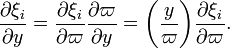$\frac{\partial\xi_i}{\partial y} = \frac{\partial\xi_i}{\partial \varpi}\frac{\partial\varpi}{\partial y} = \biggl(\frac{y}{\varpi}\biggr) \frac{\partial\xi_i}{\partial \varpi} .$

Therefore also,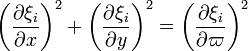$\biggl( \frac{\partial\xi_i}{\partial x} \biggr)^2 + \biggl( \frac{\partial\xi_i}{\partial y } \biggr)^2 = \biggl( \frac{\partial\xi_i}{\partial\varpi} \biggr)^2$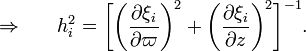$\Rightarrow ~~~~~ h_i^2 = \biggl[ \biggl(\frac{\partial\xi_i}{\partial \varpi} \biggr)^2 + \biggl(\frac{\partial\xi_i}{\partial z} \biggr)^2 \biggr]^{-1} .$

The relationship between the direction cosines when$m \ne n$ gives a key orthogonality condition. Take, for example, m = 1 and n = 2:

 ∑ γ1sγ2s = 0. s

This means that if ξ1 is orthogonal to ξ2,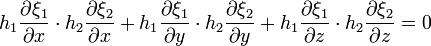$h_1 \frac{\partial\xi_1}{\partial x} \cdot h_2 \frac{\partial\xi_2}{\partial x} + h_1 \frac{\partial\xi_1}{\partial y} \cdot h_2 \frac{\partial\xi_2}{\partial y} + h_1 \frac{\partial\xi_1}{\partial z} \cdot h_2 \frac{\partial\xi_2}{\partial z}= 0$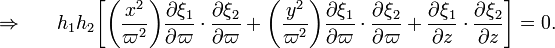$\Rightarrow ~~~~~ h_1 h_2\biggl[ \biggl( \frac{x^2}{\varpi^2} \biggr) \frac{\partial\xi_1}{\partial \varpi} \cdot \frac{\partial\xi_2}{\partial \varpi} + \biggl( \frac{y^2}{\varpi^2} \biggr) \frac{\partial\xi_1}{\partial \varpi} \cdot \frac{\partial\xi_2}{\partial \varpi} + \frac{\partial\xi_1}{\partial z} \cdot \frac{\partial\xi_2}{\partial z} \biggr] = 0 .$

Hence,

DC.02

An Example Orthogonality Condition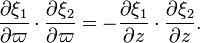$\frac{\partial\xi_1}{\partial \varpi} \cdot \frac{\partial\xi_2}{\partial \varpi} = - \frac{\partial\xi_1}{\partial z} \cdot \frac{\partial\xi_2}{\partial z} .$

### Position Vector

Employing the unit-vector transformation relations tells us that in general the position vector is,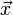$\vec{x}$ =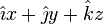$\hat\imath x + \hat\jmath y + \hat{k}z$ =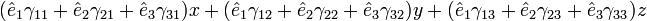$(\hat{e}_1 \gamma_{11} + \hat{e}_2 \gamma_{21} + \hat{e}_3 \gamma_{31}) x + (\hat{e}_1 \gamma_{12} + \hat{e}_2 \gamma_{22} + \hat{e}_3 \gamma_{32})y + (\hat{e}_1 \gamma_{13} + \hat{e}_2 \gamma_{23} + \hat{e}_3 \gamma_{33})z$ =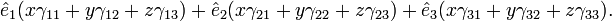$\hat{e}_1(x\gamma_{11} + y\gamma_{12} + z\gamma_{13} ) + \hat{e}_2(x\gamma_{21} + y\gamma_{22} + z\gamma_{23} ) + \hat{e}_3 (x\gamma_{31} + y\gamma_{32} + z \gamma_{33}) .$
 © 2014 - 2020 by Joel E. Tohline |   H_Book Home   |   YouTube   | Appendices: | Equations | Variables | References | Ramblings | Images | myphys.lsu | ADS |